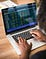“person using black and gray laptop computer” by rawpixel on Unsplash

In a previous post, we examined the fundamental tools to test for stationarity on time series using Python, one of my favorite programming languages. If we use the tools described in the article, we will very soon realise that most time series are neither stationary nor mean reverting. In this new article we are going to examine how we can test two (or more) non-stationary time series to check whether the combined value is stationary.

This is where we should introduce the notion of cointegration .

If we are able to find a stationary linear combination of several time series that are not themselves stationary, then these are called cointegrated.

We are going to see two different tests: the simpler Cointegrated Augmented Dickey-Fuller test (CADF) will be useful for pairs only, but we can apply the Johansen test to any number of time series.

# Cointegrated Augmented Dickey-Fuller Test

In the previous post, we saw how the ADF and Variance Ratio can test a given time series for mean reversion and stationarity, but we don’t know the number of units o percentage we should use to combine them into the stationary basket of elements we are looking for.

We have to be aware that just because a set of time series is cointegrating doesn’t mean that any random linear combination of the series will form a stationary basket of elements

To easily create the test we can use the procedure by Engle and Granger, which can be defined as the following steps:

1. Determine the optimal hedge ratio by running a lineal regression fit between the two series.
2. Use the hedge computed in step 1 to form a portfolio.
3. Run a stationarity test on the portfolio created in step 2.

Taking advantage of the code already written in the previous article, we can write the test easily with the help of the numpy and statsmodels libraries in the following way:

# Johansen test

In order to test for cointegration of more than two variables, we have to use the Johansen test. If we start with the linear model we already described in the previous article:

We can generalize it to the case where the variable y(t) are vectors representing multiple series, and the coefficients λ and α are actually matrices (we are also going to assume βt=0 for simplicity) and we can rewrite the equation in the following way:

Just like in the previous case with just one variable, if λ = 0 we don’t have cointegration. Let’s assume the rank of λ is r and the number of time series is n. The number of independent baskets that can be formed by different linear combinations of the cointegrating series is equal to r. And the Johansen test will calculate that number for us in two different ways, both of them based on the eigenvector decomposition of λ: the first test produces the trace statistic, and the second one produces the eigen statistic.

Here you can find a complete implementation of the Johansen Test:

Written by

## bluekiri

#### We design, deploy and manage 24/7 the cloud architecture that best suits your business needs. We ensure optimal performance of your servers and applications by identifying the most demanding processes and components of your infra and fine tuning them thanks to our super teams.

Welcome to a place where words matter. On Medium, smart voices and original ideas take center stage - with no ads in sight. Watch
Follow all the topics you care about, and we’ll deliver the best stories for you to your homepage and inbox. Explore
Get unlimited access to the best stories on Medium — and support writers while you’re at it. Just \$5/month. Upgrade